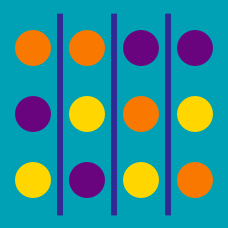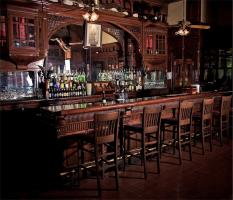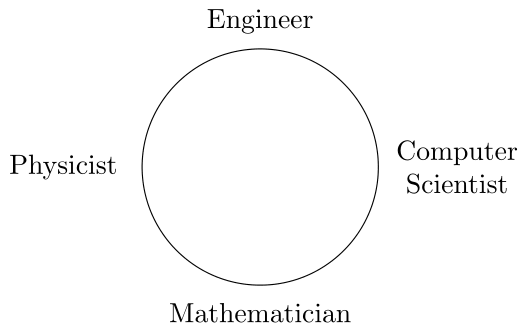Probability

# Permutations Warmup

A mathematician, a physicist, and an engineer walk into a bar. If there are 3 seats available at the bar, how many ways are there for the mathematician, physicist, and engineer to seat themselves?A mathematician, a physicist, an engineer, and a computer scientist walk into a bar. If there are 4 seats available at the bar, how many ways are for the mathematician, physicist, engineer, and computer scientist to seat themselves?

The mathematician, physicist, engineer, and computer scientist decide to move from the bar to a (circular) table. They file to the table, staying in order as they sit down and wind up seated as shown below.How many different seating arrangements at the bar will lead to the seating arrangement around the table in the image above?

Assumptions and details:

"Staying in order" means that each person has the same left and right neighbors that they had at the bar (though the person who was sitting on the left end at the bar will now be sitting to the right of the person who was sitting on the right end at the bar).

How many different ways are there for a mathematician, a physicist, an engineer, and a computer scientist to sit around a circular table if two seating arrangements are considered to be the same when each person has the same left and same right neighbor in both arrangements?

A chemist comes and joins the mathematician, physicist, engineer, and computer scientist while they are sitting at the circular table. If the chemist sits between two of the people already seated at the table, how many places can the chemist sit?

×